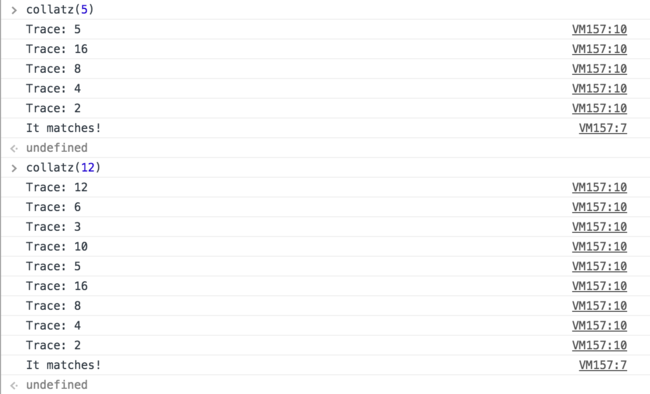# Collatz Conjecture

In this blog post we deviate a little from the ES6 parade I’ve been writing here. We dive into the Collatz Conjecture (otherwise known as the 3n + 1 conjecture) on today’s blog. The Collatz conjecture is the simplest open problem in mathematics.

## Collatz Conjecture #

The conjecture states that:

Take any positive integer n. If n is even, divide it by 2 to get n / 2. If n is odd, multiply it by 3 and add 1 to obtain 3n + 1. Repeat the process (which has been called “Half Or Triple Plus One”, or HOTPO) indefinitely. The conjecture is that no matter what number you start with, you will always eventually reach 1.

Basically if we give the number 10 the breakdown is as follows: 10 > 5 > 16 > 8 > 4 > 2 > 1 (match!). The conjecture states that every arbitrary positive integer will reach back to the number 1 after jumping through the hoops.

### Trivial Code #

So to automate most of the rudimentary math involved we will write a program to run through the possibilities until we reach the number `1` or I guess run in perpetuity if in fact the conjecture is false.

``````function collatz(num = null, trace = []) {
// Type checking and check if positive int
// Zero is defined as neither negative nor positive
if (typeof num !== 'number' || num <= 0) {
return new Error(`Invalid argument: \${num}`);
}

// Log out the trace
trace.push(num);

// Check to see if its a match
if (num === 1) {
console.log('It matches!');
return trace;
}

// Main recursion
if (num % 2 === 0) {
// even
return collatz((num / 2), trace);
} else {
// odd
return collatz(((num * 3) + 1), trace);
}
}
``````
##### Enhancements to consider #
• Dynamic programming pattern like Memoize would provide some benefits by saving a cache of the numbers already calculated so there is no need to recompute
• We could set an arbitrary limit on how deep the stack trace goes before we give up and analyze if the given `num` is in fact non-compliant with the Collatz conjecture
• Tail recursion (implemented) is only active in strict mode es2015 not es5### Importance in Computer Science #

Well… we could use the Collatz conjecture as an interview question but going beyond the most basic use case of the mathematical function `f(x)` required by the conjecture we see that the Collatz conjecture has impacts on Number Theory and Computer Science.

Gerhard Opfer, in 2011, published a paper that claims to have prove the Collatz conjecture. This proof, however, was later proven to have a flaw and was debunked.

The Collatz conjecture is similar to that of Fermat’s Last Theorem which is decidedly simple to understand but unbelievably hard to prove.

The same can be said about analyzing code.

Some minuscule codebase can have a very high complexity while other very large (cough “enterprise”) projects are very easy to implement and comprehend.